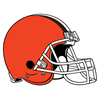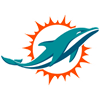DraftKings Miss Playoffs Odds
-10000
0.01 to 1Texans
50.0% implied probability

-2500
0.04 to 1Steelers
50.0% implied probability

-1600
0.06 to 1Jets
50.0% implied probability

-1400
0.07 to 1Seahawks
50.0% implied probability

-1400
0.07 to 1Bears
50.0% implied probability

-1200
0.08 to 1Commanders
50.0% implied probability

-1000
0.1 to 1Panthers
50.0% implied probability

-800
0.13 to 1Falcons
50.0% implied probability

-550
0.18 to 1Lions
50.0% implied probability

-500
0.2 to 1Patriots
50.0% implied probability

-370
0.27 to 1Raiders
50.0% implied probability

-350
0.29 to 1Cardinals
50.0% implied probability

-310
0.32 to 1Saints
50.0% implied probability

-225
0.44 to 1Giants
50.0% implied probability

-225
0.44 to 1Browns
50.0% implied probability

-215
0.47 to 1Titans
50.0% implied probability

-140
0.71 to 1Colts
50.0% implied probability

-130
0.77 to 1Broncos
50.0% implied probability

-130
0.77 to 1Jaguars
50.0% implied probability

-120
0.83 to 1Bengals
50.0% implied probability

+115
1.15 to 1Chargers
50.0% implied probability

+175
1.75 to 1Rams
50.0% implied probability

+190
1.9 to 149ers
50.0% implied probability

+200
2 to 1Cowboys
50.0% implied probability

+210
2.1 to 1Ravens
50.0% implied probability

+250
2.5 to 1Dolphins
50.0% implied probability

+300
3 to 1Vikings
50.0% implied probability

+450
4.5 to 1Packers
50.0% implied probability

+450
4.5 to 1Chiefs
50.0% implied probability

+450
4.5 to 1Buccaneers
50.0% implied probability

+900
9 to 1Eagles
50.0% implied probability

+1200
12 to 1Bills
50.0% implied probability